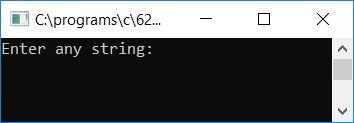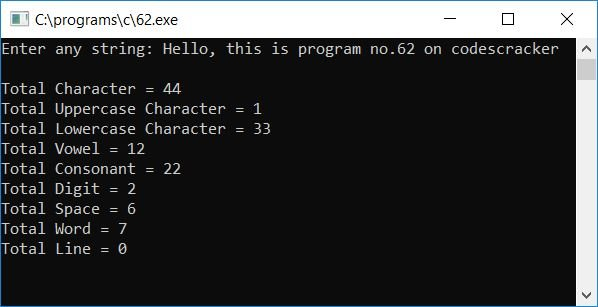# C Program to Count Consonants, Vowels, Digits, and Spaces in a String

Here we will learn about how to create a program in C that asks the user to enter any string at run-time to count the total number of:

• All characters
• Uppercase characters
• Lowercase characters
• Digits
• Vowels
• Space characters
• Words
• Lines
• Consonants

present inside the given string. Here is the program:

```#include<stdio.h>
#include<conio.h>
int main()
{
char str;
int i, count=0, countChar=0;
int countUpper=0, countLower=0, countDigit=0, countVowel=0;
int countSpace=0, countWord=0, countLine=0, countConsonant=0;
printf("Enter any string: ");
gets(str);
for(i=0; str[i]!='\0'; i++)
{
if(str[i]>=65 && str[i]<=90)
{
countUpper++;

}
else if(str[i]>=97 && str[i]<=122)
{
countLower++;
}
else if(str[i]>=48 && str[i]<=57)
countDigit++;
else if(str[i]==' ')
countSpace++;
else if(str[i]=='\n')
countLine++;
if(str[i]=='a' || str[i]=='e' || str[i]=='i' || str[i]=='o' ||
str[i]=='u' || str[i]=='A' || str[i]=='E' || str[i]=='I' ||
str[i]=='O' || str[i]=='U')
countVowel++;
if(str[i]!=' ')
count++;
else
{
if(count!=0 && str[i+1]!='\0')
countWord++;
count = 0;
}
countChar++;
}
countConsonant = (countUpper+countLower) - countVowel;
printf("\nTotal Character = %d", countChar);
printf("\nTotal Uppercase Character = %d", countUpper);
printf("\nTotal Lowercase Character = %d", countLower);
printf("\nTotal Vowel = %d", countVowel);
printf("\nTotal Consonant = %d", countConsonant);
printf("\nTotal Digit = %d", countDigit);
printf("\nTotal Space = %d", countSpace);
printf("\nTotal Word = %d", countWord+1);
printf("\nTotal Line = %d", countLine);
getch();
return 0;
}```

The program was written in the Code::Blocks IDE; therefore, here is the sample run after a successful build and run:Now you can supply any string, say Hello, this is program no.62 on codescracker and press the ENTER key to see the following output:#### Program Explained

• Declare and initialize 0 for all the variables responsible for counting the total number of
• all characters
• uppercase characters
• lowercase characters
• vowels
• consonants
• digits
• spaces
• words
• lines
• say
• countChar
• countUpper
• countLower
• countVowel
• countConsonant
• countDigit
• countSpace
• countWord
• countLine
• Now get the string input from the user at run-time.
• Create a for loop that runs from 0 to the last character of the string.
• Because the last character in any string is the null-terminated character ('\0'),
• Therefore, we have to check for each and every character present inside the string from the 0th place to before the null-terminated character.
• Create an if block inside the for loop to check the current character, that is, if it is uppercase, increment the value of the variable responsible for the uppercase character count, say countUpper, and so on.
• The ASCII code for A-Z (A to Z) is 65-90 (65 to 90). That is, A's ASCII code is 65, B's ASCII code is 66,... and Z's ASCII code is 90.
• The ASCII code for a-z is 97-122.
• The ASCII code for 0-9 is 48-57.
• After exiting from the for loop, print the value of each character one by one.

C Quiz

« Previous Program Next Program »